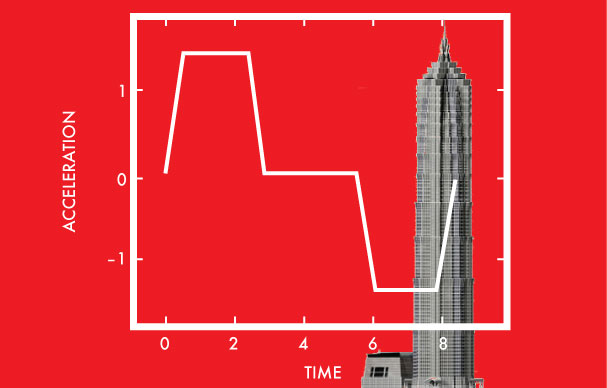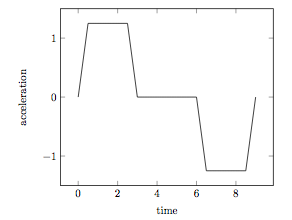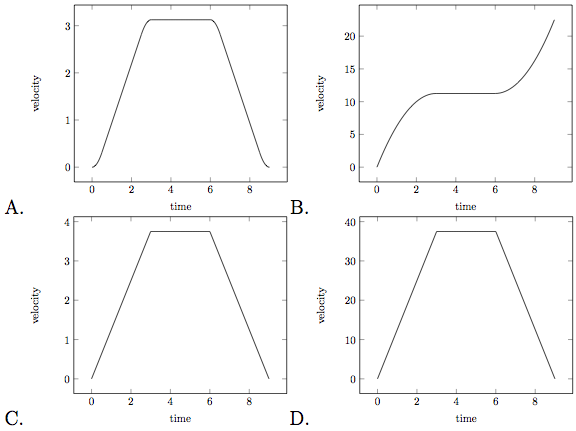# Elevator In Shanghai Tower

Calculus Level 2The Shanghai Tower has an architectural height of 632 meters, and is equipped with the world's fastest elevator which can travel at $18 m/s$. If we assume that it travels at its maximum speed, then it would take just under 36 seconds to reach the top. However, this assumption is unrealistic, as the elevator needs time to increase its velocity, i.e. accelerate.

In order to travel up several stories, the acceleration of the elevator has the following shape:Which of the following is the best graph which illustrates the velocity of the elevator?×